Grace's work on Pascal's triangle and the binomial expansion

August 8, 2002 Email from Grace to Don: Hi Don, I did some exercises in your book in Chapter 9. Attached please find my work. Also, the U of Chicago program ended two weeks ago. Bye, Grace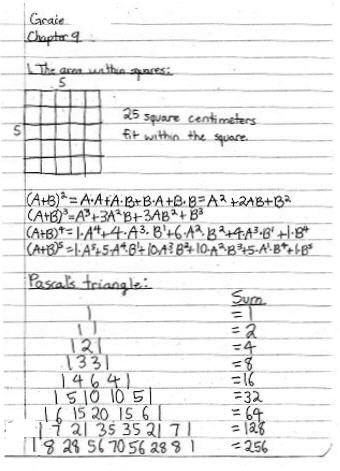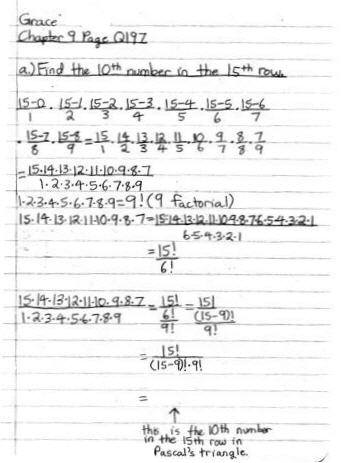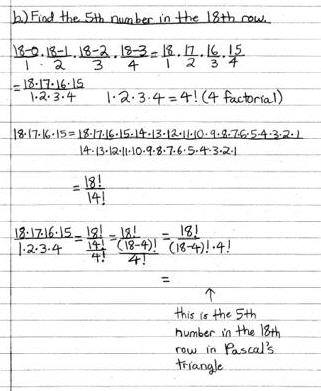Don's email to Grace Aug. 8, 2002 Hi Grace!

Great work on chapter 9, Pascal's triangle and finding the numbers in those rows!

5 things:

1. Write what number 15!/((15-9)!*9!) is equal to; and the next one, the 5th # in the 18th row.

2. Write the generalization to find the r th number in the n th row.

3. By the way, did you actually find (a+b)^3  by multiplying (a+b)*(a+b)*(a+b) ?

4. Can you write out (a+b)^8 without looking?

5. Write out the first 5 terms of  the expansion of (a+b)^n

OK?

It's a pleasure to work with you. Thank you. See you, Don

Grace's 2nd email to Don Aug. 8, 2002 Hi Don,

15!/((15-9)!*9!) is equal to 5005 and 18!/((18-4)!*4!) is equal to 3060.

I think the generalization to find the r th number in the n th row is:Attached is how I found (a+b)^3, (a+b)^8, and (a+b)^10.

I also attached some work in Chapter 8. I did it a month ago but I never sent it.

Bye, Grace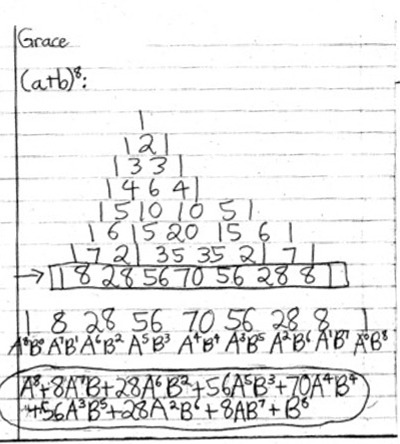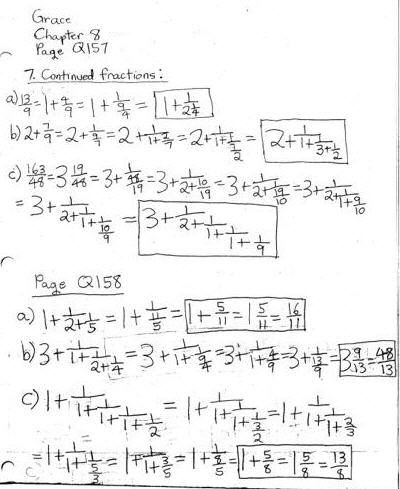See Grace's work on infinite series, cookie-sharing, bimals, graphing parabolas+

See Grace's work on multiplying two infinite series!!